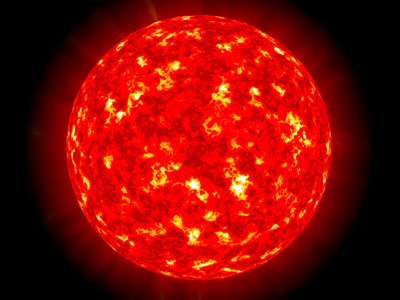The Sun is approximately 150 million km from Earth.

# Measures 3 (Easy)

You have now made it to the third of our Easy level Eleven Plus maths quizzes on Measures – well done! Just like the first two, this quiz will give you more opportunity to revise metric and Imperial measurements. For example, do you know how many kilograms there are in one-tonne? The answer is 1,000.

Imperial tonnes are a little trickier to get your head around! Here are the Imperial measures of weight:

• 1 ton (t) = 20 hundredweight (cwt)
• 1 hundredweight = 8 stones (st)
• 1 stone = 14 pounds (lbs)
• 1 pound = 16 ounces (oz)

As you can see, Imperial measures are not as easy to work with as metric ones!

Okay, now it’s time for the quiz. Take your time and be sure to understand each question before you pick your answers. Good luck!

1.
If one-yard equals 0.91 metres, to the nearest metre, how many metres are there in a furlong (1 furlong = 220 yards)?
200 metres
220 metres
242 metres
264 metres
A furlong is an eighth of a mile. You won’t come across furlongs very often, unless you are interested in horse racing
2.
An average person has around 5 litres of blood. If 1 pint = 0.57 litres, how many pints of blood are in an average body (to the nearest pint)?
3 pints
8 pints
7 pints
9 pints
To convert litres into pints, divide by 0.57: 5 ÷ 0.57 = 8.772, which we round up to 9 pints
3.
There are 4 quarts in 1 gallon. If 1 gallon = 4.55 litres, how many litres are there in 1 quart (to one decimal place)?
18.2 litres
1.1 litres
7.3 litres
14.5 litres
To work this one out, simply divide 4.55 by 4: 4.55 ÷ 4 = 1.1375, which we round down to 1.1 litres.
By the way, 1 quart is equal to 2 pints
4.
An Imperial ton = 160 stones. If 1 stone = 6.35 kg, how many kg are there in an Imperial ton?
2,500 kg
25 kg
1,016 kg
1,250 kg
This one was very easy – just multiply 160 by 6.35: 160 x 6.35 = 1,016
5.
Elsie did a sponsored walk from Land’s End to John O’Groats, a distance of 874 miles. If 1 km = 0.62 miles, how many km did Elsie walk (to the nearest km)?
1,409 km
542 km
245 km
1,410 km
To convert miles to km, divide by 0.62: 874 ÷ 0.62 = 1,409.677 which we round up to 1,410 km
6.
A recipe calls for half a pound of flour. If 1 pound = 0.454 kg, how many grams of flour are required?
454 grams
227 grams
0.454 grams
0.227 grams
If 1 pound = 0.454 kg, then half-a-pound = 0.227 kg
Next we have to convert kg to grams by multiplying by 1,000: 0.227 x 1,000 = 227 grams
7.
How many cm are there in one-quarter of a metre?
50 cm
40 cm
30 cm
25 cm
There are 100 cm in a metre, so there will be 25 cm in one-quarter of a metre: 100 ÷ 4 = 25
8.
A garden shed with a flat roof is 3.5 m long, 2.8 m wide and 2.3 m tall. What is the volume of the shed?
22.54 m³
8.6 m³
24 25 m³
25.24 m³
Volume = length × width × height = 3.5 × 2.8 × 2.3 = 22.54 m³. Make sure that for area you use square (²) and that for volume you use cube (³)
9.
The Sun is approximately 150 million km from Earth. If 1 mile equals 1.61 km, how many miles from the Earth is the Sun (to the nearest million)?
242 million miles
93 million miles
128 million miles
94 million miles
To convert km into miles, divide by 1.61: 150 ÷ 1.61 = 93.168, which we round down to 93
10.
Simon wants to paint one of the walls in his living room. The wall is 7 m wide and 2 metres high but has a doorway in it which measures 1.5 m by 2.2 m. What is the area of the wall (minus the doorway)?
14.2 m2
3.3 m2
10.7 m2
9.7 m2
First, find the area of the whole wall: 7 x 2 = 14 m2
Next, find the area of the doorway: 1.5 x 2.2 = 3.3 m2
Finally, we subtract the doorway’s area from that of the wall: 14 - 3.3 = 10.7 m2
Author:  Frank Evans